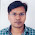### Sorting - Insertion Sort

Insertion Sort
Any basic sorting algorithm which uses comparison of elements involves a number of inversions to be made to the given array to sort it.

An Inversion in an array of numbers is any ordered pair (i,j) such that
(a[i] - a[j] )*( i - j) .
Pseudo Code

`void InsertionSort(int A[],int N){    int pos,i;    int temp;        for(pos=1; pos < n; pos++>    {         temp=A[pos];         for(j=pos;j>0;j--)         {                if(A[j-1] > temp)                {                        A[j]=A[j-1];                }                else                {                    break;                }                A[j]=temp;         }    }}`

Analysis of Insertion Sort:

The efficiency of insertion sort depends upon the distribution of the data.This is because insertion sort tries to put each of the elements in the sorted array of preceding elements.If the array is presorted , then the running time of the algorithm is O(N) because the inner for loop always breaks immediately.In the worst case, it is of O(N^2) as can be observed for each position i, the inner loop is O(i).
Hence the complexity of this algorithm is O(N^2).

Questions:

1) What is the running time of the above algorithm if all the elements in the array are equal?

Solution:O(N).Each of the Inner for loop becomes O(1).Hence the complexity O(N).

2)Suggest a modified Insertion Sort algorithm to check whether an array is sorted or not?
Give also the complexity analysis

Solution:One can prove that complexity is O(N) with out fuss.The modification is when one finds that the first swap is required just print that it is not ordered and break the loop.

1.void InsertionSort(int A[],int N)
{
int pos,i;
int temp;

for(pos=1; pos < n; pos++>
{
temp=A[pos];
for(j=pos;j>0;j--)
{
if(A[j-1] > temp)
{
A[j]=A[j-1];
}
else
{
break;
}
***---> A[j]=temp; <---***
}
}
}

I think, the marked line is misplaced.

2.Basic sorting algorithms in C: http://dailyjobquestions.com/2011/10/03/sorting-algorithms-in-c-part-1/

O(lg(n)) sorting algorithms in C: http://dailyjobquestions.com/2011/10/04/sorting-algorithms-in-c-part-2/

O(n) sorting algorithms: http://dailyjobquestions.com/2011/10/05/sorting-algorithms-in-c-part-3/

3.